# Probability Calculator

Calculate the probability of two events or a series of events using the calculator below.

%
%
%
%

## Probability Of:

Intersection of A and B:
P(A∩B):
%
Union of A and B:
P(A∪B):
%
Symmetric Difference of A and B:
P(A∆B):
%
Complement of A and B:
P((A∪B)'):
%
Complement of A:
P(A'):
%
Complement of B:
P(B'):
%
Learn how we calculated this below

## How to Calculate Probability

Probability is the likelihood of an event occurring. More specifically, it is the ratio of the event occurring to all possible outcomes.

An example of using probability is when rolling dice. You might ask yourself how to find the probability of rolling a six, for example. A more straightforward way of stating this might be, what is the chance that you’ll roll a six?

To find the probability of something happening, you need to first know the number of possible outcomes. Then, you can apply the probability formula to solve.

Continuing the dice example, there are six possible outcomes when rolling the dice. You can roll a one, two, three, four, five, or six.

### Probability Formula

This is the basic formula to solve the probability of a favorable outcome.

P(A) = number of times A can occur / number of possible outcomes

Thus, the probability of result A is equal to the number of possible times A can occur divided by the total number of possible outcomes.

So, continuing the dice example, the probability of rolling a six is thus 1/6 since there is one six on the die and six total sides.

An example to illustrate the probability of an event where the number of times the event can occur is greater than one would be the odds of drawing a spade from a deck of cards. Since there are 13 cards of each suit and 52 total cards, the probability of drawing a spade is 13/52, which simplifies to 1/4.

Our combinations and permutations calculators can help find the number of possible outcomes for a data set. This can be used to help solve the probability of an expected outcome.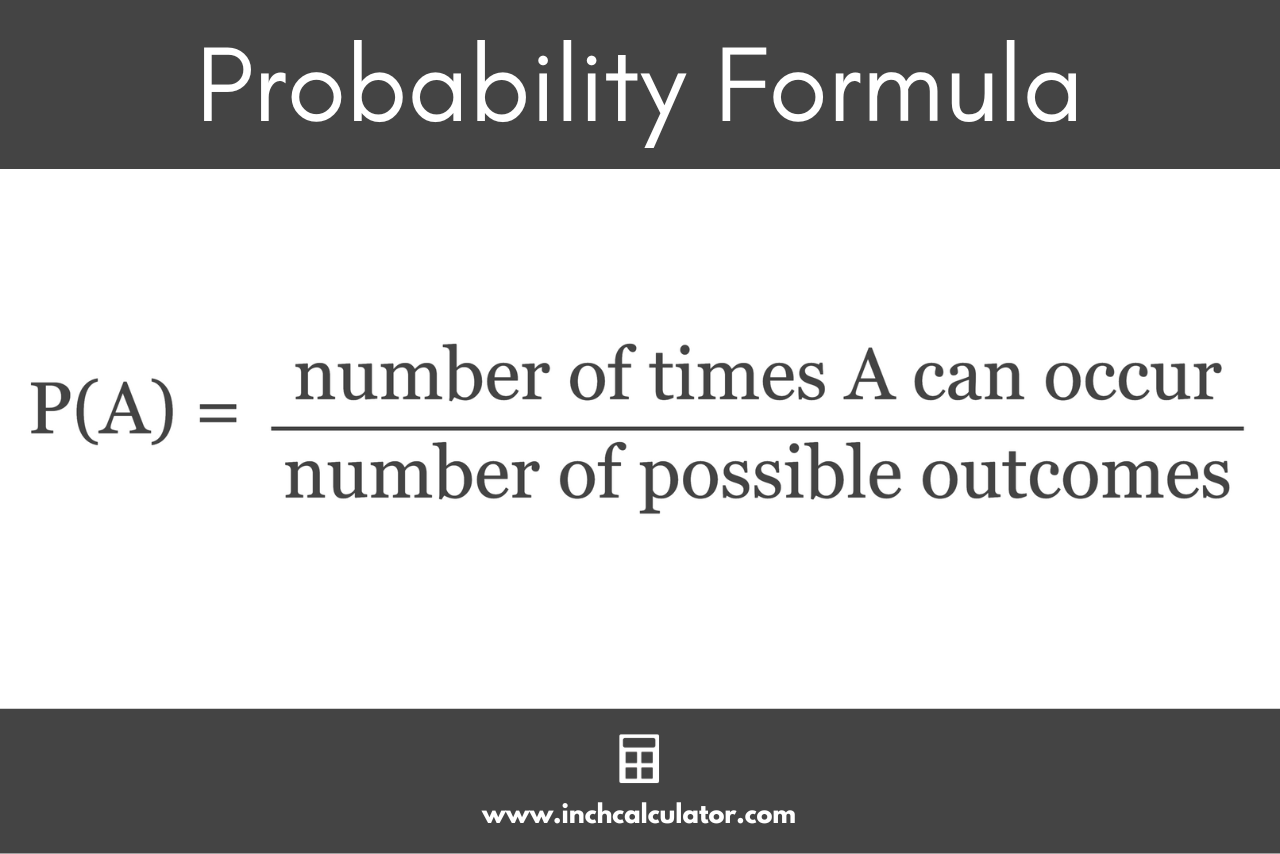### How to Find the Probability of Independent Events

Things get a little more complicated when more than one possible outcome, or event, can occur. We refer to these as independent events.

For example, you might want to know how to find the probability of getting a six when rolling two dice. In this case, we have two independent events that we need to know the likelihood of.

There are a few different variations of how independent events can occur. Let’s cover each way we could roll a six.

#### Intersection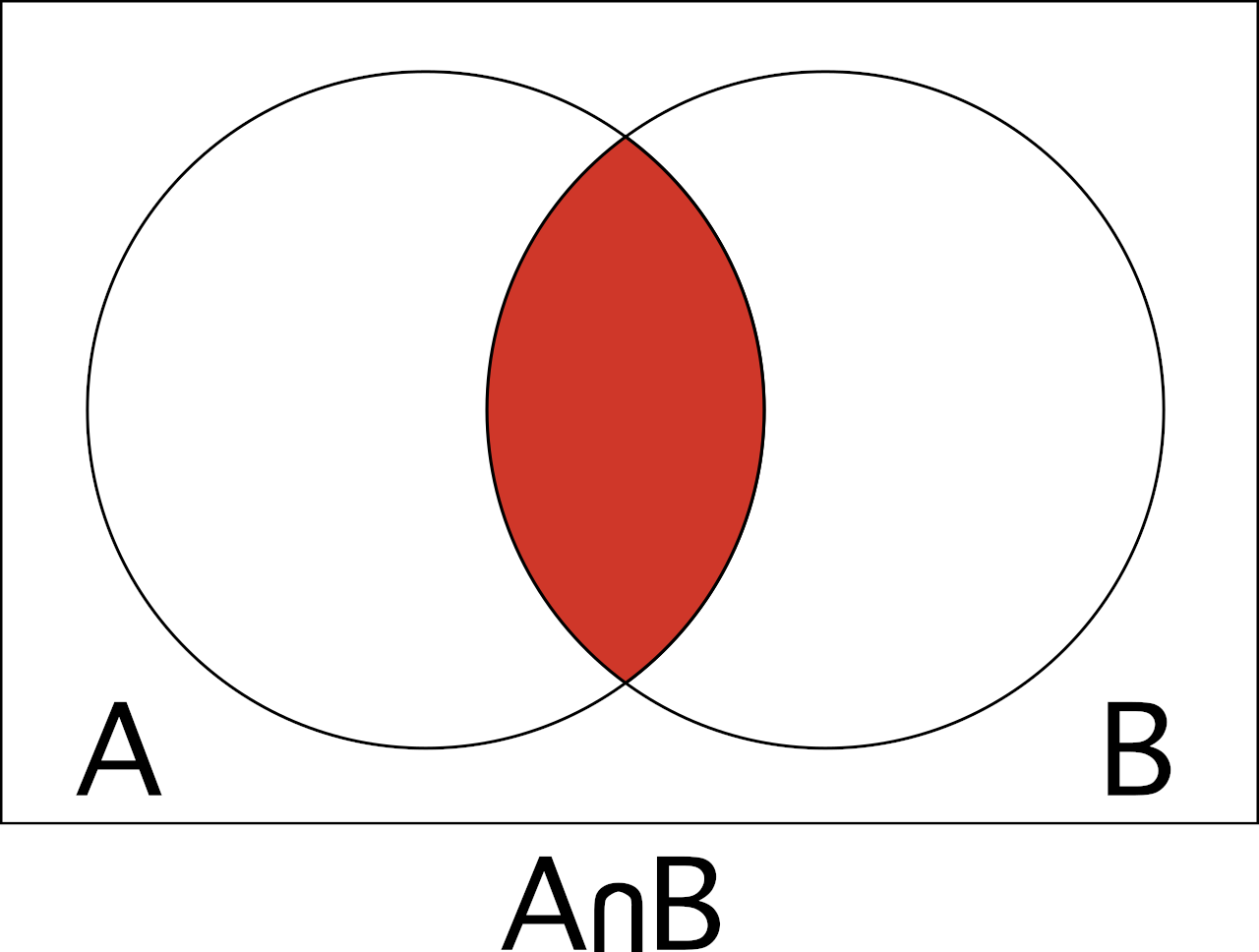In statistics, the probability of the intersection is the likelihood that both events will occur. In the dice example, this would be the chance of both dice being a six when rolling.

The intersection of events A and B is denoted A∩B, and the Venn diagram above can help visualize the intersection of events.

You can find the probability of intersection using the following formula:

P(A∩B) = P(A) × P(B)

The probability of intersection P(A∩B) is equal to P(A) times P(B).

#### Union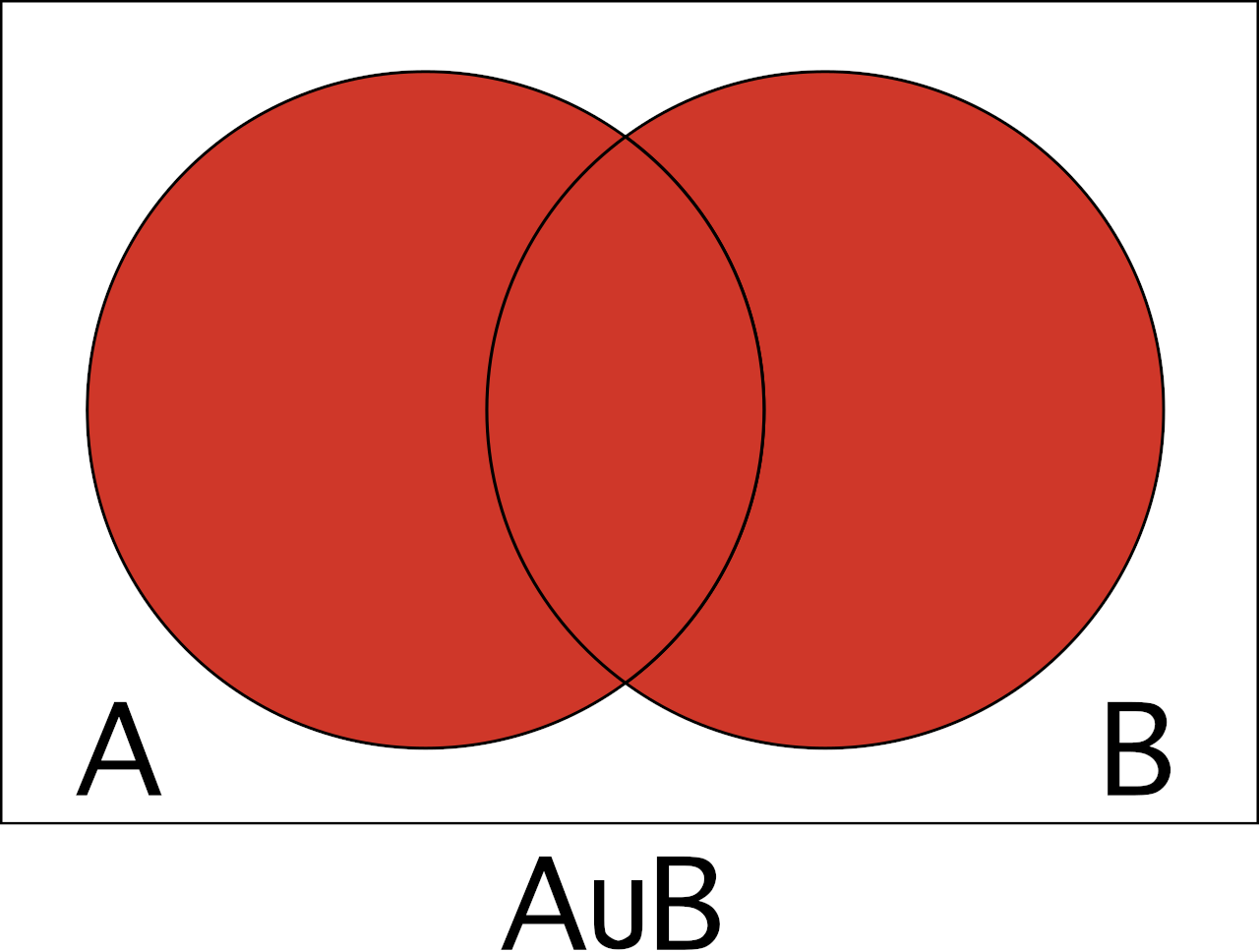The probability of the union is the likelihood that at least one of the events will occur, or both. In the dice example, this would be the chance of one of the dice being a six or both being a six.

The union of events A and B is denoted A∪B.

You can find the probability of union using the following formula:

P(A∪B) = P(A) + P(B) – P(A∩B)

The probability of union P(A∪B) is equal to P(A) plus P(B), minus the probability of intersection P(A∩B).

#### Symmetric Difference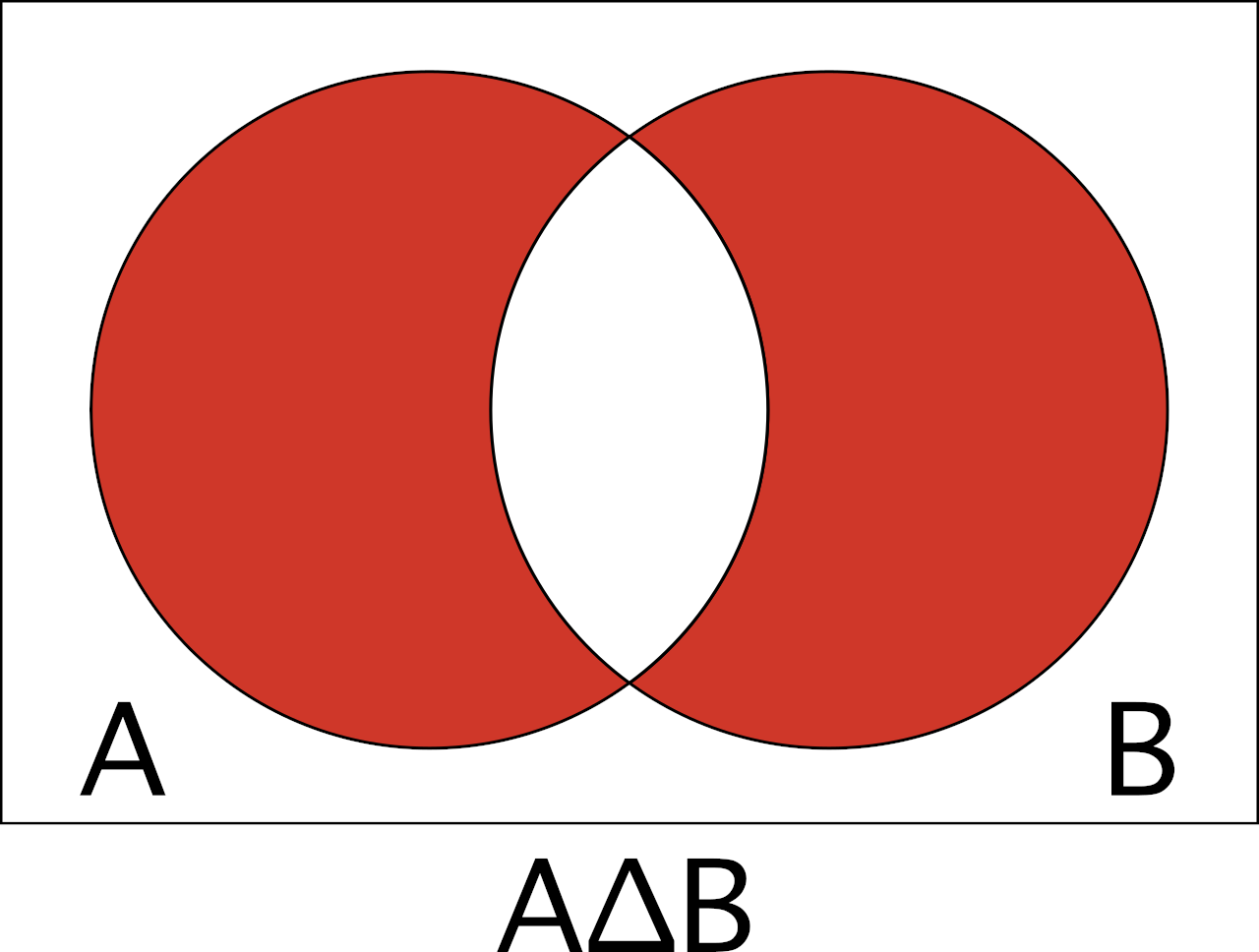The probability of the symmetric difference is the likelihood that exactly one of the events will occur, but not both. In the dice example, this would be the chance of precisely one of the dice being a six, but not both.

The symmetric difference of events A and B is denoted A∆B.

The symmetric difference is also known as the disjunctive union of two sets, and you can find the probability of it with the following formula:

P(A∆B) = P(A∪B) – P(A∩B)

The probability of the symmetric difference P(A∆B) is equal to union P(A∪B) minus the intersection P(A∩B).

#### Complement of AIn probability theory, the complement of event A is the likelihood that A does not occur. Thus, the complement of an event is effectively the chance that the event does not happen.

Continuing the dice example, the complement of rolling a six on the first dice is equal to the chance that a six is not rolled on the first dice, even if it’s rolled on the second.

Complement is denoted using an apostrophe (‘). Thus, A’ is the complement of A.

The complement can be found using the formula:

P(A’) = 1 – P(A)

The probability of the complement of A P(A’) is equal to 1 minus P(A).

#### Complement of A∪B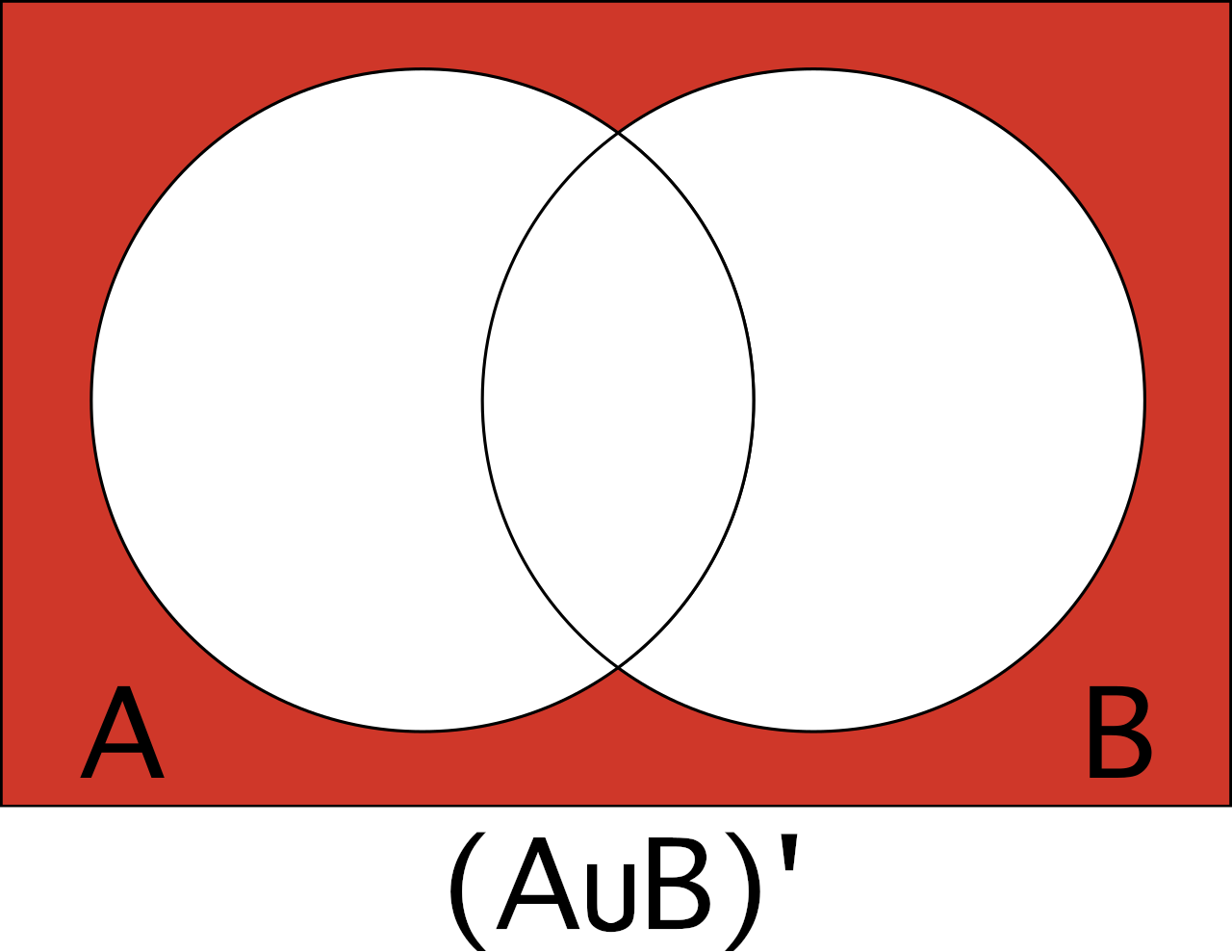The complement of the union of two events, or (A∪B)’, is the likelihood that neither event A nor event B will occur. In the dice example, this is the likelihood that neither dice is a six when rolling two dice.

P((A∪B)’) can be found using the formula:

P((A∪B)’) = 1 – P(A∪B)

The probability of the complement of A union B P((A∪B)’) occurring is equal to 1 minus the chance of A union B PA∪B).

### How to Find the Probability For a Series of Events

Sometimes you want to find the probability of an outcome occurring in a series of events. For instance, if rolling dice three times, what is the likelihood of rolling a six at least one time?

The formulas below define the probabilities of the outcome occurring at least once, every time, or never during the series.

#### Event Occurring at Least Once

P(A occurs at least once) = 1 – (1 – P(A))n

The probability of event A occurring at least once in the series of n attempts is equal to 1 minus 1 minus the probability of event A to the nth power.

#### Event Occurring Every Time

P(A occurs every time) = P(A)n

The probability of event A occurring every time in the series of n attempts is equal to the probability of event A to the nth power.

#### Event Never Occurring

P(A never occurs) = (1 – P(A))n

The probability of event A never occurring in the series of n attempts is equal to 1 minus the probability of event A to the nth power.

### How to Find the Probability For Conditional Events

So far, the formulas for single and series events have assumed the events are independent. Events are independent if one event happening does not have any impact on the probability of the other event occurring.

In the example of rolling the dice, the probability of rolling the dice and getting a six is 1/6, and the probability of rolling a six on the second roll is also 1/6. The probability of rolling a six is always 1/6 for standard dice.

However, consider the deck of cards; the probability of drawing a king from the deck is 4/52, since there are 4 kings and 52 total cards. Let’s say you draw a card that is not a king; what is the probability of drawing a king on the second draw, assuming you did not replace the first card to the deck?

The odds of drawing a king are reduced on the second draw to 4/51, since there are 4 kings and 51 total cards remaining. This is a conditional probability.

You can use Bayes’ theorem to calculate a conditional probability:

P(A|B) = P(B|A) × P(A) / P(B)

Bayes’ theorem states that the probability of event A given that event B also occurs is equal to the probability of event B given that event A also occurs times the probability of event A divided by the probability of event B.

We hope this demystifies some of the probability equations used in statistics. When in doubt, try the calculator above to see what the chances of your outcomes are!

Try our p-value calculator to calculate probabilities of a value within a distribution.